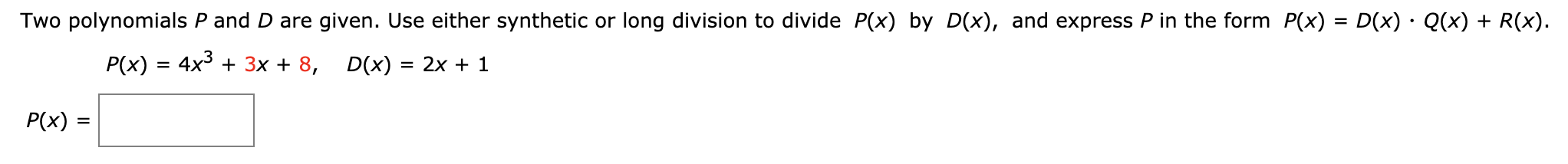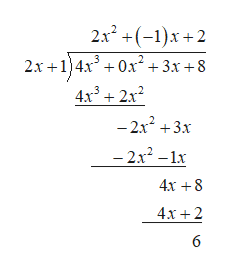# Two polynomials P and D are given. Use either synthetic or long division to divide P(x) by D(x), and express P in the form P(x) = D(x) - Q(x) + R(x)P(x) 4x33x + 8D(x) 2x + 1P(x)

Question
3 viewshelp_outlineImage TranscriptioncloseTwo polynomials P and D are given. Use either synthetic or long division to divide P(x) by D(x), and express P in the form P(x) = D(x) - Q(x) + R(x) P(x) 4x33x + 8 D(x) 2x + 1 P(x) fullscreen
check_circle

Step 1

The given polynomials are,

Step 2

Divide the polynomial P by D and express it in the following form.

Step 3

Divide the expression using the metho...help_outlineImage Transcriptionclose2x2+-1)x+2 2х +1)4х° + 0х* + 3х +8 4x32x2 -2x23x - 2x2-1x 4x 8 4x+2 6 fullscreen

### Want to see the full answer?

See Solution

#### Want to see this answer and more?

Solutions are written by subject experts who are available 24/7. Questions are typically answered within 1 hour.*

See Solution
*Response times may vary by subject and question.
Tagged in

### Calculus